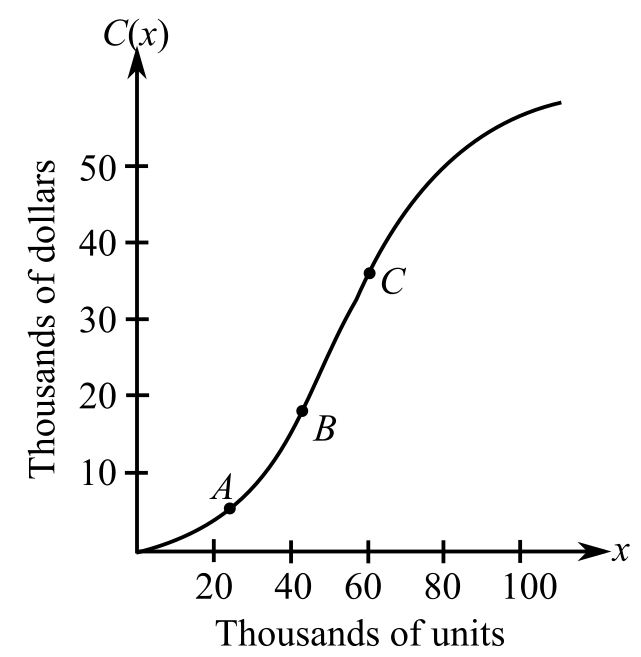Chapter 9.3, Problem 43E### Mathematical Applications for the ...

11th Edition
Ronald J. Harshbarger + 1 other
ISBN: 9781305108042

#### Solutions

Chapter
Section### Mathematical Applications for the ...

11th Edition
Ronald J. Harshbarger + 1 other
ISBN: 9781305108042
Textbook Problem

# Total cost Suppose the figure shows the total cost graph for a company. Arrange the average rates of change of total cost from A to B, B to C, and A to C from smallest to greatest and explain your choice.To determine

The average rate of change of total cost from A to B, B to C and A to C in ascending order from the total cost graph given asExplanation

Given Information:

The total cost graph given as;

Explanation:

The total cost graph given as;

If a function f(x) is defined in the interval [a,b], then its average rate of change is given by the formula,

### Still sussing out bartleby?

Check out a sample textbook solution.

See a sample solution

#### The Solution to Your Study Problems

Bartleby provides explanations to thousands of textbook problems written by our experts, many with advanced degrees!

Get Started

#### In Exercises 73-80, find the indicated limits, if they exist. 77. limxx4+1x31

Applied Calculus for the Managerial, Life, and Social Sciences: A Brief Approach

#### Sketch the graphs of the equations in Exercises 512. xy=4

Finite Mathematics and Applied Calculus (MindTap Course List)

#### If 13f(x)dx=10 and 13g(x)dx=6, then 13(2f(x)3g(x))dx= a) 2 b) 4 c) 18 d) 38

Study Guide for Stewart's Single Variable Calculus: Early Transcendentals, 8th